# Surface Areas of Cones Find the lateral areas

• Slides: 11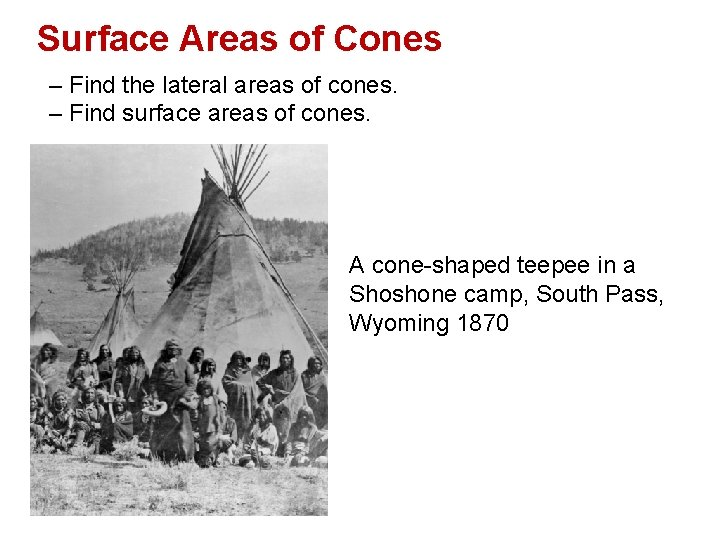Surface Areas of Cones – Find the lateral areas of cones. – Find surface areas of cones. A cone-shaped teepee in a Shoshone camp, South Pass, Wyoming 1870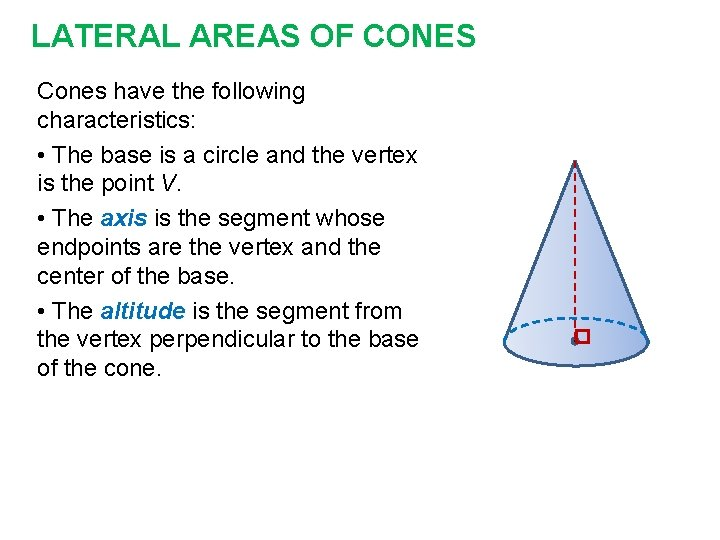LATERAL AREAS OF CONES Cones have the following characteristics: • The base is a circle and the vertex is the point V. • The axis is the segment whose endpoints are the vertex and the center of the base. • The altitude is the segment from the vertex perpendicular to the base of the cone.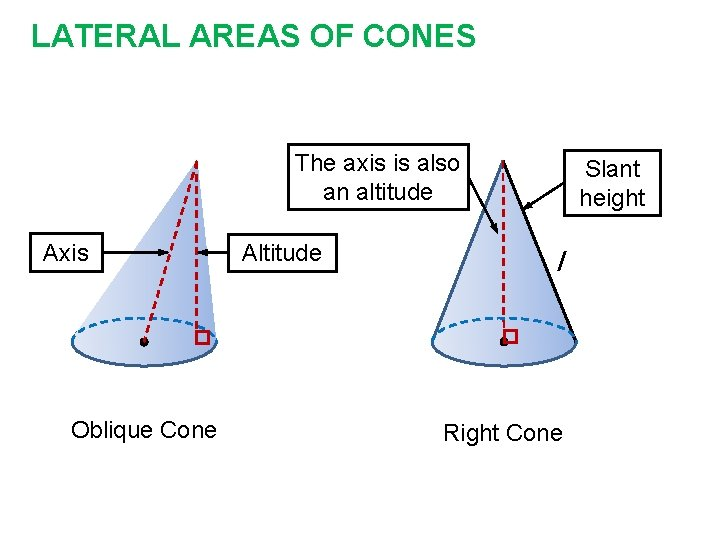LATERAL AREAS OF CONES The axis is also an altitude Axis Oblique Cone Altitude Slant height l Right ConeLATERAL AREAS OF CONES We can use the net for the cone to derive the formula for the lateral area. r l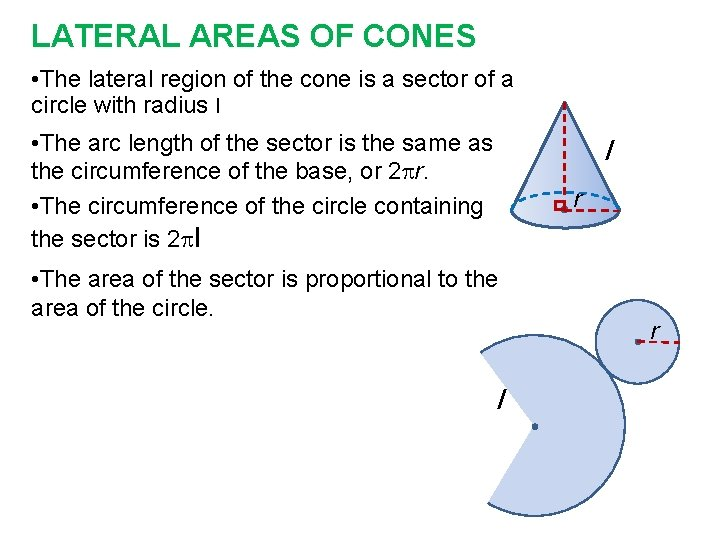LATERAL AREAS OF CONES • The lateral region of the cone is a sector of a circle with radius l • The arc length of the sector is the same as the circumference of the base, or 2 r. • The circumference of the circle containing the sector is 2 l l r • The area of the sector is proportional to the area of the circle. l r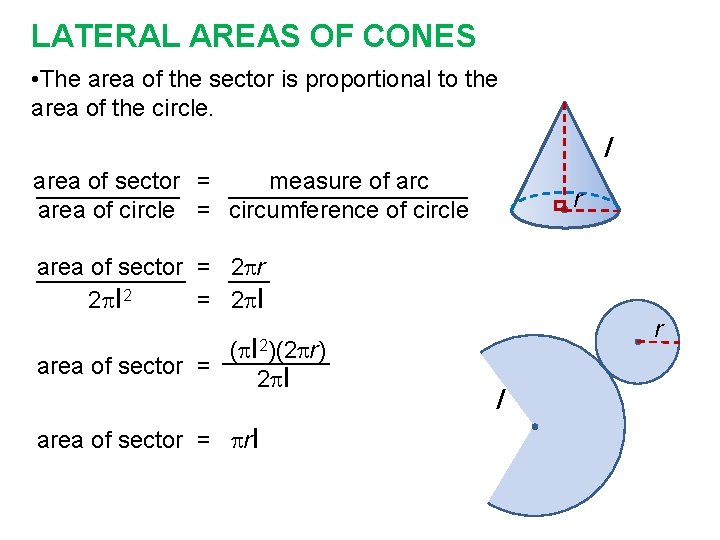LATERAL AREAS OF CONES • The area of the sector is proportional to the area of the circle. l area of sector = measure of arc area of circle = circumference of circle r area of sector = 2 r 2 l 2 = 2 l ( l area of sector = 2 l r 2)(2 r) area of sector = rl l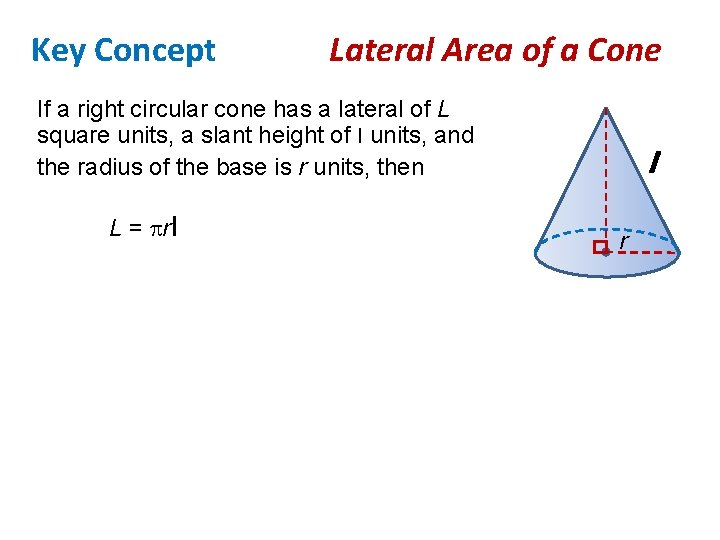Key Concept Lateral Area of a Cone If a right circular cone has a lateral of L square units, a slant height of l units, and the radius of the base is r units, then L = rl l r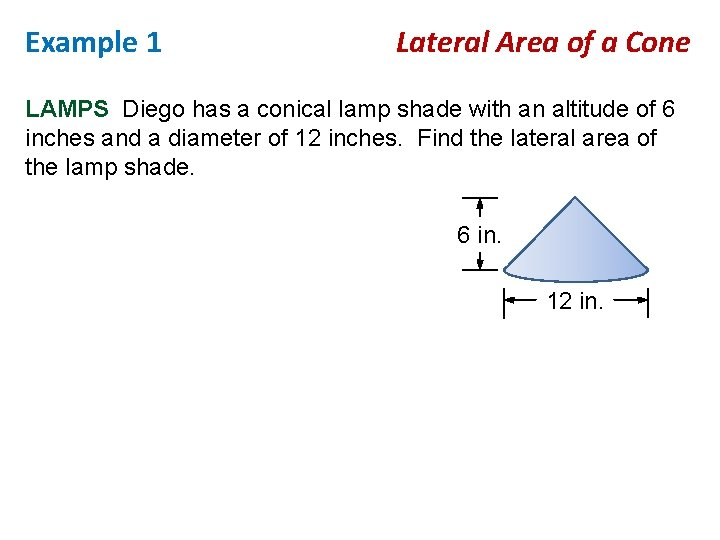Example 1 Lateral Area of a Cone LAMPS Diego has a conical lamp shade with an altitude of 6 inches and a diameter of 12 inches. Find the lateral area of the lamp shade. 6 in. 12 in.SURFACE AREAS OF CONES To find the surface area of a cone, add the area of the base to the lateral area.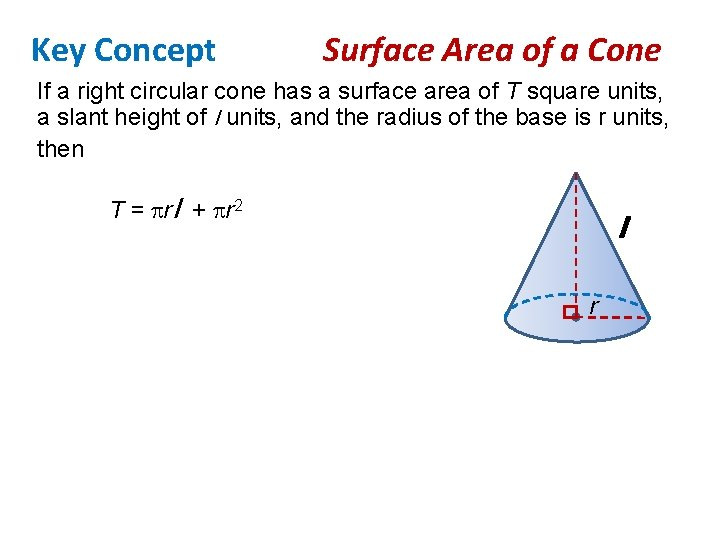Key Concept Surface Area of a Cone If a right circular cone has a surface area of T square units, a slant height of l units, and the radius of the base is r units, then T = rl + r 2 l r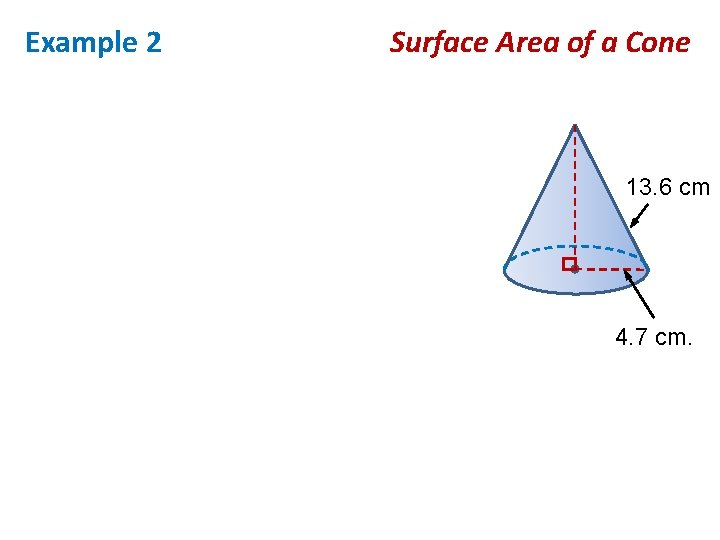Example 2 Surface Area of a Cone 13. 6 cm 4. 7 cm.